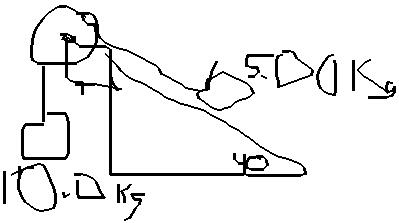Question# Two Packing crates of masses 10.0 kg and 5.00kg are connected by alight string that passes over a frictionless pulley as in Figure.

Other
ANSWEREDTwo Packing crates of masses 10.0 kg and 5.00kg are connected by alight string that passes over a frictionless pulley as in Figure. The 5.00-kg crate lies on a smooth incline of angle 40.0 degrees. Find the acceleration of the 5.00-kg crate and the tension in the string.2020-11-02
Let's analyze this problem one chunk at the time. We'll Let:
m = 5kg
M = 10kg
For the 10kg mass:
Mg - tension = a(m + M)
the 5kg mass:
$$\displaystyle\text{tension}-{m}{g}{\sin{{\left(\theta\right)}}}={a}{\left({m}+{M}\right)}$$
Since both equations equal a(m+M), you can set them equal:
$$\displaystyle{M}{g}-\text{tension}=\text{tension}-{m}{g}{\sin{{\left(\theta\right)}}}$$
$$\displaystyle{M}{g}={2}\text{tension}-{m}{g}{\sin{{\left(\theta\right)}}}$$
$$\displaystyle\text{tension}={\frac{{{M}{g}+{m}{g}{\sin{{\left(\theta\right)}}}}}{{{2}}}}$$
After you find tension, you can substitute it back into one of the earlier equations to find a.
$$\displaystyle{M}{g}-\text{tension}={a}{\left({m}+{M}\right)}$$
$$\displaystyle{a}={\frac{{{M}{g}-\text{tension}}}{{{m}+{M}}}}$$